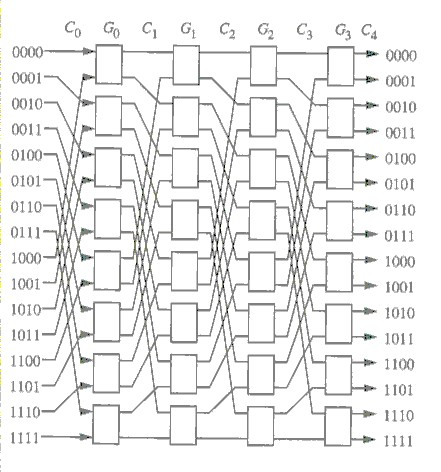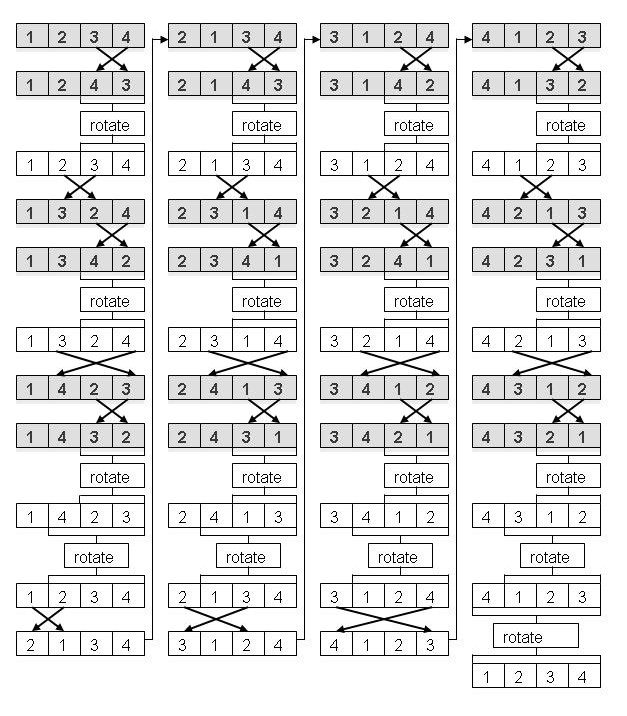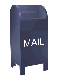## Calculating Permutations and Job Interview Questions

The permutation of a set is the number of ways that the items in the set can be uniquely ordered. For example, the permutations of the set { 1, 2, 3} are { 1, 2, 3}, { 1, 3, 2}, { 2, 1, 3}, { 2, 3, 1}, { 3, 1, 2} and { 3, 2, 1}. For N objects, the number of permutations is N! (N factorial, or 1 * 2 * 3 * ... N).

Aside from theoretical interest in set theory, permutations have some practical use. Permutations can be used to define switching networks in computer networking and parallel processing (see Figure 1). Permutation also used in a variety of cryptographic algorithms.Figure 1, An Omega permuation network, from Interconnection Networks: an engineering approach. by Duato, Yalamanchili and Ni, Morgan Kaufmann, 2003, Pg. 32

### Permutation Algorithms and Job Interview Questions

I wrote this web page as a result of a job interview. This may seem like a strange chain of motivation. Let me explain...

If you take a look at my resume you'll see that I've worked at a number of different companies, so I've done a fair amount of interviewing. There was even a time when I interviewed for jobs just to keep in practice.

Those interviewing a candidate for a software engineering position understandably would like to discover whether the candidate can write software. One way to determine this is to ask about past projects. What did the project involve? What did the candidate contribute to the project? What was the architecture of the software system? What problems came up and how were they solved?

For most of my career, interviews for software engineering positions followed this format. The first time I encountered a radically different interview style was when I interviewed with Microsoft in June of 2000.

A Microsoft recruiter looking for people for their compiler group called me up and persuaded me to travel to Redmond, Washington to interview with the Microsoft compiler group. I spent a day talking to the main compiler group and another half a day talking to Microsoft Research.

As one rotates through the members of the group that you are interviewing with at Microsoft, you are asked again and again to go to a white board and write algorithms in the form of C/C++ code. After a morning of this, I was taken out to lunch by a group member. We went to a good Italian restaurant and I thought that I would have a respite from problem solving. I was wrong. Over lunch he asked me one of those famous Microsoft thought puzzles. After lunch it was back to white board problems. The people I talked to at Microsoft asked very little about the work I had done in the past.

I don't think quickly on my feet and I get "stage fright". Even for algorithms that I've written many times, like in-order binary tree traversal, I've frozen and been unable to recall the algorithm.

The Microsoft style interview seems to be spreading. I've encountered this style of interview at nVidia and at two EDA start-up companies (at nVidia a guy who looked a bit like the rocker Joe Jackson walked into the conference room, told me his name and immediately started firing programming problems at me). To date I've never been offered a job after an interview like this.

On a number of occasions I've pointed out to the interviewer that if they want to know whether I can write code, they can look at bearcave.com where I've published thousands of lines of C++ and Java code. For what ever reason, the interviewers have been unwilling to accept this as evidence of my software engineering abilities. Perhaps the reason is that, deprived of their white board problems, they don't know what else to ask. Interviewing people for an job is difficult. The interviewer must decide what characteristics are important and what questions will illuminate these characteristics. The interviewer may also have to interact with the interviewee on a person-to-person level, which they may be uncomfortable with. It is a lot easier asking someone to write an algorithm on the white board.

"Write the code on the white board" interviewers must confront the problem of picking an algorithm that is small enough to fit on a white board, complex enough to be difficult and solvable in the time allotted to the interview. In some cases the result is a "tricky" algorithm. In one case the answer was an algorithm with a time complexity of summation of N (e.g., 1 + 2 + 4 + ... N), which one would never use in practice since there were better algorithms which did not meet the artificial constraints of the interviewer's problem.

I spent many years working on compiler design and implementation. A modern compiler could be regarded as a collection of complex algorithms, some of which are heuristic. So for years I've been collecting and reading books on software algorithms. This came in handy when, during a phone interview, I was asked what the time complexity of a permutation was (e.g., N!). The interviewer then asked me how I would implement permutation and I said that one would use a recursive swapping algorithm. Since this is difficult to describe over the phone, they asked me to mail them the algorithm. The last algorithm on this web page (the lexicographic permutation algorithm, inspired by Bryan Flamig's algorithm, was the result).

The fact that I knew the answer to the questions about permutation does not mean that I am, or am not, a good software engineer. These are remarkably tricky recursive algorithms. They are not easy to figure out and they are not like most algorithms I've worked with. A hiring decision made on such a basis is rather arbitrary.

Permutations algorithms are interesting and they are, on rare occasion, useful. But they should not be used as questions in job interviews. Here, for the use and study of the next victim of such pointless questions, are three permutation algorithms, with links to a few more.

### Permutation Algorithms

Permutation sets are usually calculated via recursion. Recursive algorithms have several common characteristics: the algorithms are powerful, they can be difficult to understand and as a result, can be difficult to develop. There are a variety of methods for recursively calculating permuations. I've listed three here:

1. A recursive permutation algorithm closely based on a Java algorithm published on Alexander Bogomolny web page Counting And Listing All Permutations

2. A permutation algorithm based on a string permutation algorithm from the course notes of the University of Exeter's Computational Physics class (PHY3134) (I was not able to identify an author).

3. An ordered (lexicographic) permuation algorithm. This algorithm is based on a a permutation algorithm from the book Practical Algorithms in C++ by Bryan Flamig, John Wiley and Sons, 1995

Additional lexicographic permutation algorithm can be found on Alexander Bogomolny's permutations web page, including a lexicographic permutation algorithm based on one invented by Edsger W. Dijkstra.

The following recursive permutation code is based on Alexander Bogomolny's algorithm.

#### Alexander Bogomolyn's unordered permutation algorithm

```
#include <stdio.h>

void print(const int *v, const int size)
{
if (v != 0) {
for (int i = 0; i < size; i++) {
printf("%4d", v[i] );
}
printf("\n");
}
} // print

void visit(int *Value, int N, int k)
{
static level = -1;
level = level+1; Value[k] = level;

if (level == N)
print(Value, N);
else
for (int i = 0; i < N; i++)
if (Value[i] == 0)
visit(Value, N, i);

level = level-1; Value[k] = 0;
}

main()
{
const int N = 4;
int Value[N];
for (int i = 0; i < N; i++) {
Value[i] = 0;
}
visit(Value, N, 0);
}

```

The result, for N = 4, is shown below. If the sets are sorted it become clear that the result is correct.

```   1   2   3   4
1   2   4   3
1   3   2   4
1   4   2   3
1   3   4   2
1   4   3   2
2   1   3   4
2   1   4   3
3   1   2   4
4   1   2   3
3   1   4   2
4   1   3   2
2   3   1   4
2   4   1   3
3   2   1   4
4   2   1   3
3   4   1   2
4   3   1   2
2   3   4   1
2   4   3   1
3   2   4   1
4   2   3   1
3   4   2   1
```

#### University of Exeter algorithm

The Exeter permuations are in close to sorted order. If a sorting step (using bubble sort) were added to produce lexicographic ordering, the Exeter algorithm may still be faster than mine, which uses a recursive set of rotations.

```
#include <stdio.h>

void print(const int *v, const int size)
{
if (v != 0) {
for (int i = 0; i < size; i++) {
printf("%4d", v[i] );
}
printf("\n");
}
} // print

void permute(int *v, const int start, const int n)
{
if (start == n-1) {
print(v, n);
}
else {
for (int i = start; i < n; i++) {
int tmp = v[i];

v[i] = v[start];
v[start] = tmp;
permute(v, start+1, n);
v[start] = v[i];
v[i] = tmp;
}
}
}

main()
{
int v[] = {1, 2, 3, 4};
permute(v, 0, sizeof(v)/sizeof(int));
}

```

The Exeter algorithm is building what is, in effect, a temporary set on the recursive stack.

The output of this code shown below. The sets that are out of lexicographic order are marked. It appears that bubble sort would reorder the permutations in N steps.

```   1   2   3   4
1   2   4   3
1   3   2   4
1   3   4   2
1   4   3   2
1   4   2   3 <==
2   1   3   4
2   1   4   3
2   3   1   4
2   3   4   1
2   4   3   1
2   4   1   3 <==
3   2   1   4
3   2   4   1
3   1   2   4
3   1   4   2
3   4   1   2
3   4   2   1
4   2   3   1
4   2   1   3 <==
4   3   2   1
4   3   1   2 <==
4   1   3   2
4   1   2   3 <==
```

#### An Ordered Lexicographic Permutation Algorithm based on one published in Practical Algorithms in C++

The next permutation algorithm produces the permutations in lexicographic (or sorted) order. Here we assume that there is a defined ordering between the elements in the set, which can define a sorting order. For example, in the set {1, 2, 3} the sorting order is 1 < 2 < 3 or in the set {a b c}, a < b < c.

The algorithm is diagrammed below for a set of four objects. The algorithm recursively calculates the permutations of larger and larger subsets of the set (e.g., a set of two elements, a set of three elements...}. There is a cost incurred when the permuation is produced in lexicographic order. After each recursive step, the subset is rotated back into the original order in the preceeding stage. The time complexity of this reordering appears to be similar to the cost that would be incurred by sorting the final permutation set, so this reordering may not be justified.Figure 2

A C++ implementation of this algorithm is shown below. The source code can be downloaded here

```
#include <stdio.h>

void print(const int *v, const int size)
{
if (v != 0) {
for (int i = 0; i < size; i++) {
printf("%4d", v[i] );
}
printf("\n");
}
} // print

void swap(int *v, const int i, const int j)
{
int t;
t = v[i];
v[i] = v[j];
v[j] = t;
}

void rotateLeft(int *v, const int start, const int n)
{
int tmp = v[start];
for (int i = start; i < n-1; i++) {
v[i] = v[i+1];
}
v[n-1] = tmp;
} // rotateLeft

void permute(int *v, const int start, const int n)
{
print(v, n);
if (start < n) {
int i, j;
for (i = n-2; i >= start; i--) {
for (j = i + 1; j < n; j++) {
swap(v, i, j);
permute(v, i+1, n);
} // for j
rotateLeft(v, i, n);
} // for i
}
} // permute

```

back to Miscellaneous Software